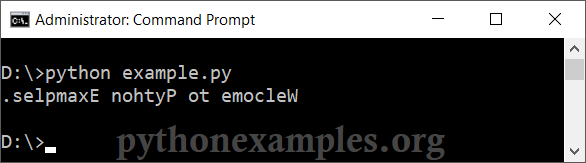# Python Program to Reverse String

## Reverse String in Python

There is no standard function from Python to reverse a string, But we can use other methods to reverse a string, through slicing, for loop, etc.

In this tutorial, we will go through the above mentioned methods to reverse a String in Python.

### Example 1: Reverse String using Slicing

To reverse a string in Python, we will use slicing. Specifically, we will use `[::-1]`. Here we have not provided the start and end, therefore slicing would happen from start to end of the string. -1 would make the slicing happen in reverse, i.e., from end to start. And `str[::-1]` would result in a reversed string.

Python Program

``````#the string
str = "Welcome to Python Examples."
#reverse string using slicing
reversed = str[::-1]
#print reversed string
print(reversed)``````
Run

OutputExample: Python Reverse String

### Example 2: Reverse String using For Loop

In this example, we will reverse a string using Python For Loop. With for loop, we iterate over characters in string and append each character at the front of a new string(initially empty). By the end of the for loop, we should have a reversed string.

Python Program

``````#the string
str = "Welcome to Python Examples."

#reverse string using for loop
reversed = '' #store reversed string char by char
for c in str:
reversed = c + reversed  # appending chars in reverse order

#print reversed string
print(reversed)``````
Run

Output

``````D:\>python example.py
.selpmaxE nohtyP ot emocleW``````

### Example 3: Reverse String using While Loop

In this example, we use a Python While Loop statement, and during each iteration, we decrement the length. We use this length variable as index to extract values from string. With every step of the loop, we extract a character from decreasing end of the string.

Python Program

``````#the string
str = "Welcome to Python Examples."

#reverse string using while loop
reversed = '' #store reversed string char by char
length = len(str) - 1
while length >= 0:
reversed = reversed + str[length]
length = length - 1

#print reversed string
print(reversed)``````
Run

Output

``````D:\>python example.py
.selpmaxE nohtyP ot emocleW``````

### Example 4: Reverse String using List.reverse()

Following is the sequence of steps to reverse a string using List.reverse().

1. Convert string to list of characters.
2. Reverse the List.
3. Join the list items.

Python Program

``````#the string
str = "Welcome to Python Examples."

#convert string to list of chars
str_list = list(str)

#reverse the list
str_list.reverse()

#join the list items
reversed = ''.join(str_list)

#print reversed string
print(reversed)``````
Run

Output

``````D:\>python example.py
.selpmaxE nohtyP ot emocleW``````

### Summary

In this tutorial of Python Examples, we learned how to reverse a String in Python language with the help of well detailed example programs.